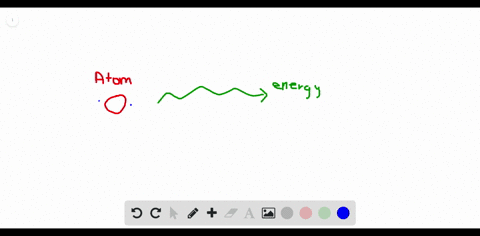### Problem 79

00:18
Problem 79
Problem 80University of Miami
Problem 78

Data for chromium's four naturally occuring isotopes are
provided in Table 4.7 . Calculate chromium's atomic mass.

Total atomic mass $=51.996$ amu.

## Discussion

You must be signed in to discuss.

## Video Transcript

now we'll work on problem 78 from chapter four. This problem were given some data for some isotopes of chromium for four isotopes and were asked to calculate Crimeans atomic Mass. So the way we do this is we simply need for each isotope to multiply the abundance by the mask and and together the result for each. So first, um, in this table we have chromium 50 which has a percent abundance of 4.35 So we divide that percent abundance by 100 begin 0.435 to represent percent as a decimal. Then we multiply by the mass of this isotope, which is 49.946 Now, we're gonna do the same for each of the other three isotopes and add them altogether. So the second isotope was most abundant at 83.79%. We divide by 100 to get their 0.8379 and multiply it by the mass of this I put I said hope, which is 51.9 for one. We do the same thing for third isotope 0.950 is our abundance and desperate form multiplied by the mass. We continue on the second line, little finalized. That hope has an abundance of 2.36% which we divide by 100 multiplied by 50 3.939 And when we some all of these up we get a mass of 51.996 atomic mass units. That's the atomic mass for